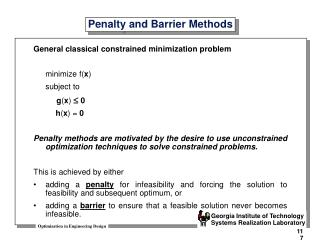# Penalty and Barrier Methods - PowerPoint PPT PresentationDownload PresentationPenalty and Barrier Methods

Penalty and Barrier Methods
Download Presentation## Penalty and Barrier Methods

- - - - - - - - - - - - - - - - - - - - - - - - - - - E N D - - - - - - - - - - - - - - - - - - - - - - - - - - -
##### Presentation Transcript

1. General classical constrained minimization problem minimize f(x) subject to g(x) 0 h(x) = 0 Penalty methods are motivated by the desire to use unconstrained optimization techniques to solve constrained problems. This is achieved by either adding a penalty for infeasibility and forcing the solution to feasibility and subsequent optimum, or adding a barrier to ensure that a feasible solution never becomes infeasible. Penalty and Barrier Methods

2. Two approaches exist in the choice of transformation algorithms: 1) Sequential penalty transformations 2) Exact penalty transformations Penalty Methods Penalty methods use a mathematical function that will increase the objective for any given constrained violation. General transformation of constrained problem into an unconstrained problem: min T( x ) = f( x ) + r P( x ) k where • f( x ) is the objective function of the constrained problem • r is a scalar denoted as the penalty or controlling parameter k • P( x ) is a function which imposes penalities for infeasibility (note that P( ) is x controlled by r ) k • T( x ) is the (pseudo) transformed objective

3. Sequential Penalty Transformations are the oldest penalty methods. Also known as Sequential Unconstrained Minimization Techniques (SUMT) based upon the work of Fiacco and McCormick, 1968. Sequential Penalty Transformations

4. Two major classes exist in sequential methods: 1) First class uses a sequence of infeasible points and feasibility is obtained only at the optimum. These are referred to as penalty function or exterior-point penalty function methods. 2) Second class is characterized by the property of preserving feasibility at all times. These are referred to as barrier function methods or interior-point penalty function methods. Two Classes of Sequential Methods General barrier function transformation T(x) = y(x) + r B( x ) k where B( x ) is a barrier function and r the penalty parameter which is supposed to go to k zero when k approaches infinity. Typical Barrier functions are the inverse or logarithmic, that is: m m å -1 B( x ) = –  g ( x ) or B( x ) = –  ln[–g ( x )] å i i i=1 i=1

5. Some prefer barrier methods because even if they do not converge, you will still have a feasible solution. Others prefer penalty function methods because • You are less likely to be stuck in a feasible pocket with a local minimum. • Penalty methods are more robust because in practice you may often have an infeasible starting point. However, penalty functions typically require more function evaluations. Choice becomes simple if you have equality constraints. (Why?) What to Choose?

6. Theoretically, an infinite sequence of penalty problems should be solved for the sequential penalty function approach, because the effect of the penalty has to be more strongly amplified by the penalty parameter during the iterations. Exact transformation methods avoid this long sequence by constructing penalty functions that are exact in the sense that the solution of the penalty problem yields the exact solution to the original problem for a finite value of the penalty parameter. However, it can be shown that such exact functions are not differentiable. Exact methods are newer and less well-established as sequential methods. Exact Penalty Methods

7. Typically, you will encounter sequential approaches. Various penalty functions P(x) exist in the literature. Various approaches to selecting the penalty parameter sequence exist. Simplest is to keep it constant during all iterations. Always ensure that penalty does not dominate the objective function during initial iterations of exterior point method. Closing Remarks